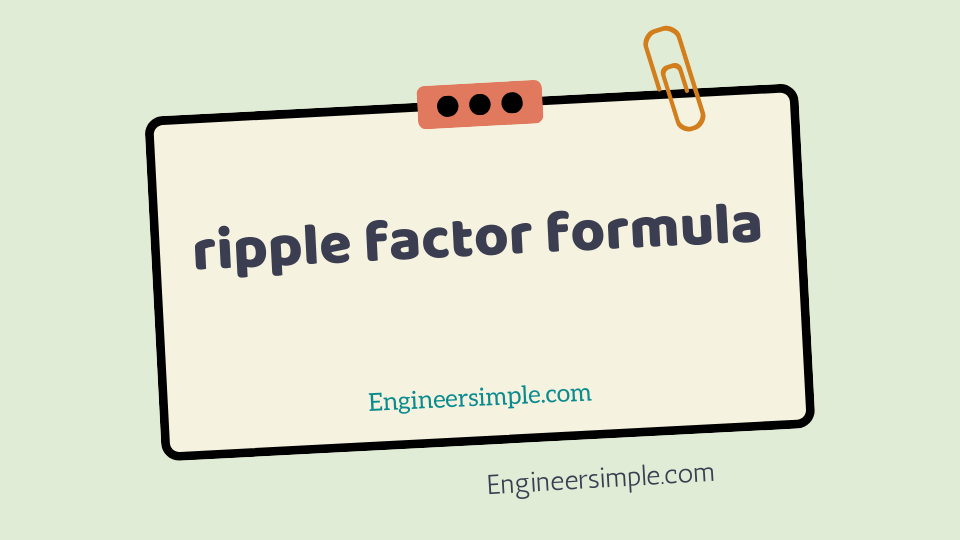ripple factor formula - Engineer Simple

#The output of a rectifier consists of a d.c. component and as a.c. component (also
known as ripple). The a.c. component is undesirable and accounts for the pulsations in
the rectifier output. The effectiveness of a rectifier depends upon the magnitude of a.c.
component in the output; the smaller this component, the more effective is the rectifier.
The ratio of r.m.s. value of a.c. component to the d.c. component in the rectifier
output is known as factor i.e.

Therefore, it is very important in deciding the effectiveness of a
rectifier. The smaller the ripple factor, the lesser the effective a.c. component and hence
more effective is the rectifier.

Mathematical analysisThe output current of a rectifier contains d.c. as well as
a.c. component. The undesired a.c. component has a frequency of 100H z (i.e.
double the supply frequency 50 Hz) and is called the (See Fig. 1). It is a
fluctuation superimposed on the d.c. component.
By definition, the effective (i.e. r.m.s.) value of total load current is given by: